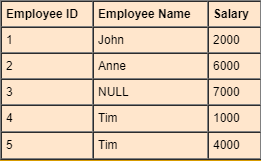Select PageThis SQL tutorial focuses on the SQL Group Functions in SQL Server, and provides explanations, examples and exercises. For this lesson’s exercises use this link.

## Definition

SQL Server Group Functions operate on sets of rows to give one result per group, for example :## SQL Server Common Group Functions

 Function Description Syntax SUM Returns the total sum ```SUM(salary) -- Result: 20000 ``` MIN Returns the lowest value ```MIN (salary) -- Result: 1000 ``` MAX Returns the highest value ```MAX(salary) -- Result: 7000 ``` AVG Returns the average value ```AVG(salary) -- Result: 4000 ``` COUNT (*) Returns the number of records in a table ```COUNT(*) -- Result: 5 ``` COUNT (column) Returns the number of values (NULL values will not be counted) of the specified column ```COUNT(name) -- Result: 4 ``` COUNT (DISTINCT column) Returns the number of distinct values ```COUNT(DISTINCT name) -- Result: 3 ```

* Results based on the illustration mentioned above

## SQL Server GROUP Functions and NULL

• In SQL Server, All Group functions ignore NULL values. For example: the average salary is calculated based on the rows in the table where a valid value is stored (the total salary divided by the number of employees receiving a salary).
• In SQL Server You can use the ISNULL function to force group functions to include NULL values, in the following example the average is calculated based on all rows in the table, regardless of whether null values are stored in the salary column (the total salary divided by the total number of rows in the table):
```SELECT       AVG(ISNULL(salary,0))
FROM         Employees
```

## The SQL Server GROUP BY Clause

```SELECT       column_name , group_function(column_name)
FROM         table_name
WHERE        condition
GROUP BY     column_name
```

So far, each group function described here has treated the table as one large group of data. In most cases you need to divide the table into smaller groups, instead of getting the average salary of all employees in Employees table, you would rather see, for example, the average salary grouped by each department (what is the average salary of the HR department, IT department and so on).

You can use the SQL Server GROUP BY clause to divide the rows in a table into groups. Then you can use the group functions to retrieve summary information for each group.

```SELECT      department_id , AVG(salary)
FROM        employees
GROUP BY    department_id
```

## A Few Guidelines

All columns in the SQL Server SELECT clause that are not group functions must be in the GROUP BY clause

When specifying group functions (AVG)  in a SELECT clause, alongside with other individual items (department_id), you must include a GROUP BY clause. In the GROUP BY clause you must specify these individual items (department_id in this case)  otherwise an error will be generated.

The SQL Server GROUP BY columns don’t have to be in the SQL Server SELECT clause

It is absolutely possible to group by different columns, but not to specify these columns in the SQL Server SELECT clause (however the result will not be meaningful). In this example we’re displaying the average salary for each departments without displaying the respective department numbers.

```SELECT      AVG(salary)
FROM        employees
GROUP BY    department_id
```

You can list more than one column after the SQL Server GROUP BY clause

Sometimes you need to see the result for groups within groups, for example: in each department there are various positions (administrative positions, clerk, maintenance employees and so on). While the operations carried out so far were meant to display the average of each department, the query specified below allows seeing the average of each department, divided by position type:

```SELECT      department_id , job_id , AVG(salary)
FROM        employees
WHERE       department_id IN (50, 80, 90)
GROUP BY    department_id , job_id
```

Using the SQL Server WHERE clause, you can exclude rows before dividing them into groups.

For example, if you need to display the average salary only for departments 50, 80, and 90:

```SELECT      department_id , AVG(salary)
FROM        employees
WHERE       department_id IN (50, 80, 90)
GROUP BY    department_id
```

You cannot use the SQL Server WHERE clause to restrict groups

As seen in the last example, using the SQL Server WHERE clause you can restrict rows before dividing them into groups. However, it is not possible to specify group functions in a SQL Server WHERE clause, as that would result in an error.

The departments where the average salary is higher than 5000:

``` SELECT      department_id , AVG(salary)
FROM        employees
WHERE       AVG(salary) > 5000
GROUP BY    department_id

(error)
```

## The HAVING Clause

The SQL Server HAVING clause allows filtering of aggregated results produced by the SQL Server GROUP BY clause. In the same way you used SQL Server WHERE clause to restrict rows, you use the SQL Server HAVING clause to restrict groups.

```SELECT       column_name , group_function(column_name)
FROM         table_name
WHERE        condition
GROUP BY     column_name
HAVING       condition
```

The departments where the average salary is higher than 5000:

```SELECT      department_id , AVG(salary)
FROM        employees
GROUP BY    department_id
HAVING      AVG(salary) > 5000
```

In a single query you can use both SQL Server HAVING and WHERE clauses. Out of departments 80, 50, and 90, the departments where the average salary is higher than 5000:

```SELECT      department_id , AVG(salary)
FROM        employees
WHERE       department_id IN (50, 80, 90)
GROUP BY    department_id
HAVING      AVG(salary) > 5000
```

You can filter based on another group function than the one that appears in a SQL Server SELECT statement:

``` SELECT      department_id , AVG(salary)
FROM        employees
WHERE       department_id IN (50, 80, 90)
GROUP BY    department_id
HAVING      MAX(salary) > 5000
```